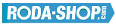# Remote ControlManufacturer Product Image Item Name Price### 61803

Thin Section Bearings Chrome Steel Open(No Shields) Dimension d=17.00 Dimension D=26.00 Dimension B=5.00 Dinamic Load Rating=2233 Static Load Rating=1456 Weight=0.009gr. Speed limit Grease=26.0 Speed limit Oil=30.0
inside diameter = 17.00
outside diameter = 26.00
width = 5.00
€4.54### 61803-2RS

Thin Section Bearings Chrome Steel Rubber Seals on Both sides Dimension d=17.00 Dimension D=26.00 Dimension B=5.00 Dinamic Load Rating=2233 Static Load Rating=1456 Weight=0.009gr. Speed limit Grease=26.0 Speed limit Oil=30.0
inside diameter = 17.00
outside diameter = 26.00
width = 5.00
€5.02### 61803-2Z

Thin Section Bearings Chrome Steel Metallic Shields on both sides Dimension d=17.00 Dimension D=26.00 Dimension B=5.00 Dinamic Load Rating=2233 Static Load Rating=1456 Weight=0.009gr. Speed limit Grease=26.0 Speed limit Oil=30.0
inside diameter = 17.00
outside diameter = 26.00
width = 5.00
€4.54### 61804-2RS

Thin Section Bearings Chrome Steel Rubber Seals on Both sides Dimension d=20.00 Dimension D=32.00 Dimension B=7.00 Dinamic Load Rating=4015 Static Load Rating=2462 Weight=0.018gr. Speed limit Grease=21.0 Speed limit Oil=25.0
inside diameter = 20.00
outside diameter = 32.00
width = 7.00
€4.84### 61804-2Z

Thin Section Bearings Chrome Steel Metallic Shields on both sides Dimension d=20.00 Dimension D=32.00 Dimension B=7.00 Dinamic Load Rating=4015 Static Load Rating=2462 Weight=0.018gr. Speed limit Grease=21.0 Speed limit Oil=25.0
inside diameter = 20.00
outside diameter = 32.00
width = 7.00
€4.84### 61805-2RS

Thin Section Bearings Chrome Steel Rubber Seals on Both sides Dimension d=25.00 Dimension D=37.00 Dimension B=7.00 Dinamic Load Rating=4303 Static Load Rating=2932 Weight=0.024gr. Speed limit Grease=18.0 Speed limit Oil=21.0
inside diameter = 25.00
outside diameter = 37.00
width = 7.00
€4.84### 61805-2Z

Thin Section Bearings Chrome Steel Metallic Shields on both sides Dimension d=25.00 Dimension D=37.00 Dimension B=7.00 Dinamic Load Rating=4303 Static Load Rating=2932 Weight=0.024gr. Speed limit Grease=18.0 Speed limit Oil=21.0
inside diameter = 25.00
outside diameter = 37.00
width = 7.00
€4.84### 61900

Thin Section Bearings Chrome Steel Open(No Shields) Dimension d=10.00 Dimension D=22.00 Dimension B=6.00 Dinamic Load Rating=2695 Static Load Rating=1273 Weight=0.01gr. Speed limit Grease=34.0 Speed limit Oil=41.0
inside diameter = 10.00
outside diameter = 22.00
width = 6.00
€5.00### 61900-2RS

Thin Section Bearings Chrome Steel Rubber Seals on Both sides Dimension d=10.00 Dimension D=22.00 Dimension B=6.00 Dinamic Load Rating=2695 Static Load Rating=1273 Weight=0.01gr. Speed limit Grease=34.0 Speed limit Oil=41.0
inside diameter = 10.00
outside diameter = 22.00
width = 6.00
€4.24### 61900-2Z

Thin Section Bearings Chrome Steel Metallic Shields on both sides Dimension d=10.00 Dimension D=22.00 Dimension B=6.00 Dinamic Load Rating=2695 Static Load Rating=1273 Weight=0.01gr. Speed limit Grease=34.0 Speed limit Oil=41.0
inside diameter = 10.00
outside diameter = 22.00
width = 6.00
€4.24